# Analog Circuits - Online Test

Q1.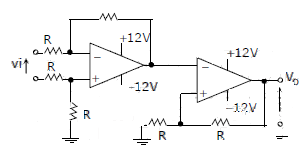The CORRECT transfer characteristic is

Explaination / Solution:

It is a Schmitt trigger and phase shift is zero.

Q2. The circuit shown is a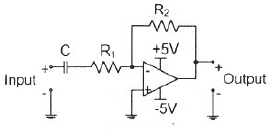Explaination / Solution:Q3. The voltage gain Av of the circuit shown below is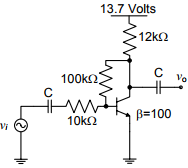Explaination / Solution:Q4. In the circuit shown below what is the output voltage out (Vout) in Volts if a silicon transistor Q and an ideal op-amp are used?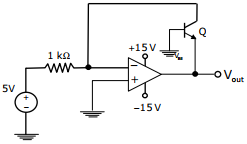Explaination / Solution:
No Explaination.

Q5. In the circuit shown below the op-amps are ideal. Then Vout in Volts is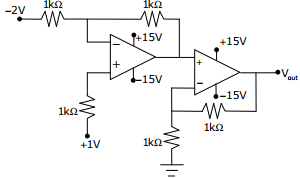Explaination / Solution:
No Explaination.

Q6.
The filters F1 and F2 having characteristics as shown in Figures (a) and (b) are connected as shown in Figure (c).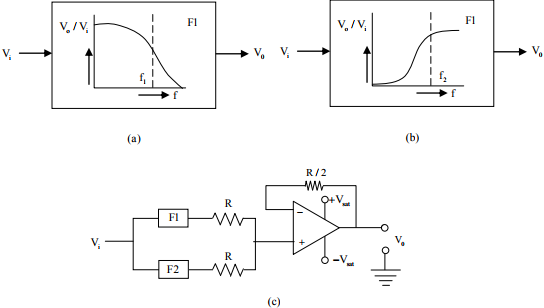The cut-off frequencies of F1 and F2 are f1 and f2 respectively. If f1 < fthe resultant circuit exhibits the characteristics of a

Explaination / Solution:
No Explaination.

Q7. The saturation voltage of the ideal op-amp shown below is ±10V. The output voltage v0 of the following circuit in the steady-state isExplaination / Solution: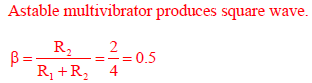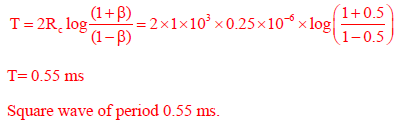Q8. A temperature in the range of −40˚ C to 55˚ C is to be measured with a resolution of 0.1˚ C. The minimum number of ADC bits required to get a matching dynamic range of the temperature sensor
Explaination / Solution: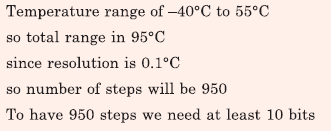Q9. A 2-bit flash Analog to Digital Converter (ADC) is given below. The input is 0 ≤ VIN ≤ 3 Volts. The expression for the LSB of the output B0 as a Boolean function of X2X1, and X0 isExplaination / Solution: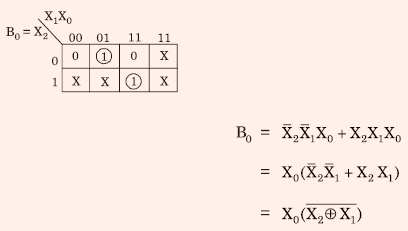Q10. For the circuit shown below, taking the opamp as ideal, the output voltage Vout in terms of the input voltages V1 , V2 and V3 is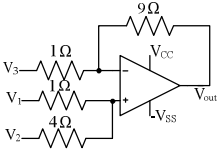Explaination / Solution: